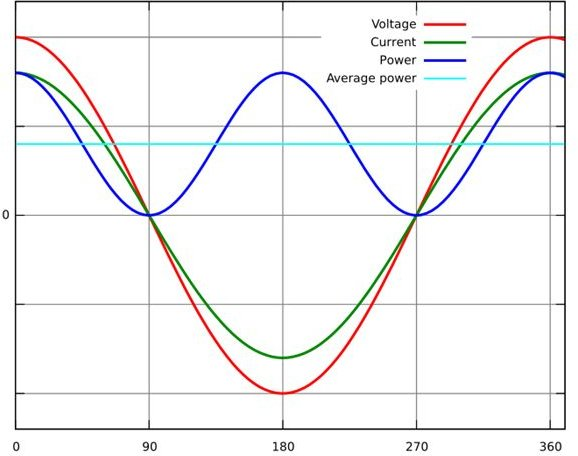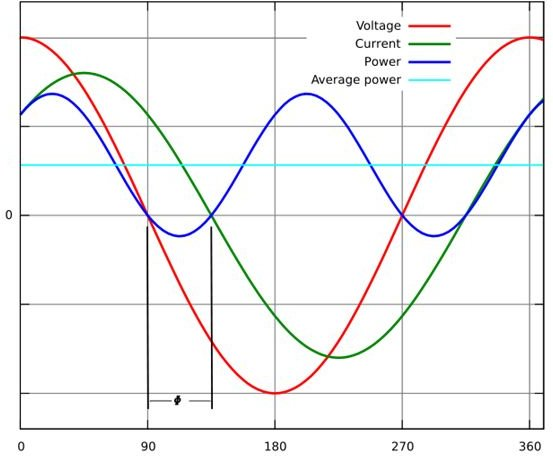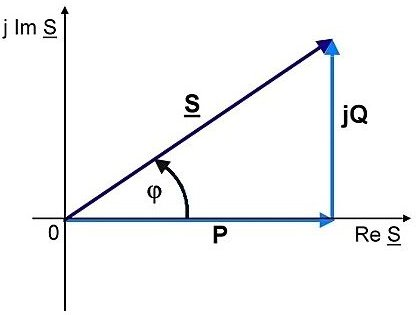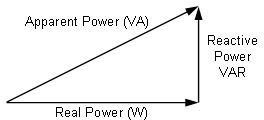# Reverse Real and Reactive Power Phasors

## Real / Active Power (P):

This type of power flow is best illustrated by considering a simple resistor connected to a single phase AC supply of V volts. The resistor will take a current I and will take electrical energy from the supply and convert into heat. The wave forms of voltage and current are in phase I =V/ R. In this there is no leading or lagging power factor.

The power waveform is obtained by multiplying the Voltage and Current together. You will see that V and I are both negative the power wave form will be positive. In fact, the power flow is always positive.

Positive power flow means that power is taken from the supply and converted by the resistor into a power output. This type of power is called active power. Active power flow occurs when V and I are in phase.

Active power is more often called kilowatt (KW) and reactive power called KiloVars (KVAr).

From the diagram below (click the image to enlarge and use the Back button in your browser to return to this page): Instantaneous and average power calculated from AC voltage and current with a unity power factor (φ=0, cosφ=1). Since the blue line is above the axis, all power is real power consumed by the load.

## Real Power:## Reactive Power (Q):

Imagine a coil which has zero resistance, wound around a steel core. The device is connected to an AC supply V volt and will take an AC current I amps. Consider the current wave form at the instant the current is zero. As the current starts to increase, it will create a magnetic field in the steel core. The strength of this field increases as the current builds up.

During the quarter cycle between, the electrical current transfers energy from the supply and stores it in the magnetic field. In the next quarter cycle the current falls to zero, and the magnetic field along with the stored energy collapses.

Since there is no power output, the only place it can go is back into the supply, so the device now feeds power back into the supply.

In the next quarter cycle, the current builds up again and power flow is supply – device and the process repeat itself.

Power is alternately positive and negative as energy is stored and discharged. So the power wave form is first positive and then negative. To create this power flow the current lags behind the voltage by 90o.

This to and fro flow of electrical power is called reactive and is caused by power devices that can store energy in a magnetic field (motors, fluorescent lamps, etc.).

Reactive power Q = V × I

When V and I are at 90oto each other the reactive power reverses every quarter cycle.

The main electrical services comprising a typical load would be electrical motor, lighting, and heating devices. These entire loads will draw KW from the supply because they all provide power out puts, but motors and florescent lighting have coils and steel cores so they also require KVAr. A typical load will require both KW and KVAr simultaneously from the generator. To do this the load current lags the voltage at an angle Φ, where Φ is somewhere between (0 -90).

Real Power P = V × I in phase.

Reactive Power Q = V × I at 90 degrees.

So P= VI cos Φ KW

Q= VI sin Φ KVAr.

From the diagram below:

Temporary Storage of Energy:

Instantaneous and average power calculated from AC voltage and current with a zero power factor (φ=90, cosφ=0). The blue line shows all the power is stored temporarily in the load during the first quarter cycle and returned to the grid during the second quarter cycle, so no real power is consumed.

Releasing the Stored Energy:

Instantaneous and average power calculated from AC voltage and current with a lagging power factor (φ=45, cosφ=0.71). The blue line shows some of the power is returned to the grid during the part of the cycle labeled φ.

Power Reversal

Reverse Reactive power to Real Power:

As the reactive power is mainly due to the inductive effect, the current lags behind the voltage causing the lagging power factor to reduce the effect and reversal of reactive power to the active power, so we introduce a capacitance. The capacitance performs the opposite action in what is known as the capacitive effect {the current leads the voltage causing the leading power factor). Thus the net effect is nullified to obtain the required real power.

## Reactive Power## Apparent Power (S):

You will notice from the phasor diagram that there are 3 Volt –amp products.

1. V I Cos Φ –Active Power (P).
2. V I Sin Φ –Reactive Power (Q).
3. VI – Apparent Power the volts times the actual current, a power but not a new type. It is just the combination of P and Q and is called the Apparent Power (S).

Apparent power (S) = Volt Current (amps).

Unit of Apparent Power – VA.

Power Factor:

If you known the KVA in a system you may need to know how much of it consist of KW. This is indicated by the power factor of the system.

Power factor = KW / KVA = VI Cos Φ / VI = Cos Φ.

KW = (KVA Cos Φ).

For a three phase power system

• Active Power P =√3 VI CosΦ.
• Recative Power Q =√3 VI SinΦ.
• Apparent Power S =√3 VI.
1. (Apparent Power)2 = (Real power)2 + (Reactive power)2
2. (Real Power) = (Apparent Power) cos(Φ)
3. (Reactive power) = (Apparent Power) sin(Φ)

Normally copper loss of a transformer depends on the current and iron loss on voltage, hence total transformer loss depends on Volt-Ampere (VA) and not on the phase angle between voltage and current, i.e. it is independent of load power factor.

This is why the rating of a transformer is in KVA and not in KW.

Image Credit:

en.wikipedia.org/wiki/Electrical_resistance

en.wikipedia.org/wiki/Power_factor

en.wikipedia.org/wiki/AC_power

## Power Triangle: